# QlikView Documents

QlikView documentation and resources.

Announcements
Document boards are being consolidated, this board no longer allows NEW documents READ MORE

# Inter-Record Functions

cancel
Showing results for
Did you mean:Master

## Inter-Record Functions

Inter-Record Functions:

These functions are used when a value from previously loaded records of data is needed for the evaluation of the current record.

For Example  ,will Take Sample Table  and Perform all the Inter - Record Functions ,

```Table:
Variant, Customer, Sales
S1, Wilson, 12
S2, Johnny, 55
S3, Jenny, 6
S4, Mary, 4
S4, Rob, 33
S5, Dave, 44
];```

(Copy and paste below code into the edit script window and reload then at Front end

remember to sort dimension and expression by load order)

 1.Field Functions:

A) Field Index :

Syntax:    fieldindex( fieldname, value )

• Returns the position of the field value in the field fieldname (by loadorder). If value cannot be found among the field values of the field fieldname, 0 is returned. Fieldname must be given as a string value,

e.g. the field name must be enclosed by single quotes.

This is like vLookup.

Example:From this above table Field Index ,

FieldIndex('Customer','Jenny')

Output: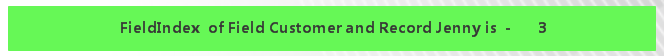B) Field Value :

Syntax:    fieldvalue( fieldname, n )

• Returns the field value found in position n of the field field name (by load order). Field name must be given as a string value, e.g. the field name must be enclosed by single quotes. The first field value is returned for n=1. If n is larger than the number of field values,NULL is returned.

Example:From this above table Field value ,

FieldValue('Customer',2)

Output:C) Field Value Count:

Syntax:    fieldvaluecount ( fieldname )

• Returns the number of distinct values in a field. Fieldname must be given as a string (e.g. a quoted literal).

Example:From this above table Field value Count ,

FieldValueCount('Customer')

Output: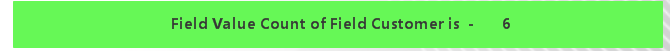2.Above Function:

Syntax:  above( [ total ] expression [, offset [,n ]] )

• Returns the value of expression evaluated with the chart's dimension values as they appear on the row above the current row within column segment in a table or, in the case of image charts, in the chart's straight table equivalent.
• On the first row of a column segment a null value will be returned,as there is no row above.

From the Above Data Following will be the Outputs,

Sum(Sales):Above(Sales):3.Below Function:

Syntax:  below( [ total ] expression [, offset [,n ]] )

• Returns the value of expression evaluated with the chart's dimension values as they appear on the row below the current row within a column segment in a table or, in the case of image charts, in the chart's straight table equivalent.
• On the last row of a column segment a null value will be returned,as there is no row below.

From the Above Data Following will be the Outputs,

Sum(Sales):Below(Sales):4.Before Function:

Syntax:  before( [ total ] expression [, offset [,n ]] )

• Returns the value of expression evaluated with a pivot table’s dimension values as they appear in the column before the current column within a row segment in the pivot table. This function returns NULL in all chart types except pivot tables.
• In the first column of a row segment a null value will be returned, as there is no column before it.

Sum(Sales):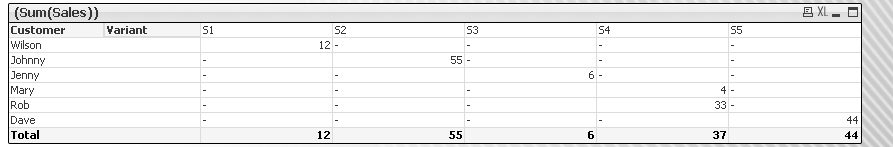Before(Sales):5.After Function:

Syntax:  after( [ total ] expression [, offset [,n ]] )

• Returns the value of expression evaluated with a pivot table’s dimension values as they appear in the column after the current column within a row segment in the pivot table. This function returns NULL in all chart types except pivot tables.
• In the last column of a row segment a null value will be returned, as there is no column after it.

Sum(Sales):Before(Sales):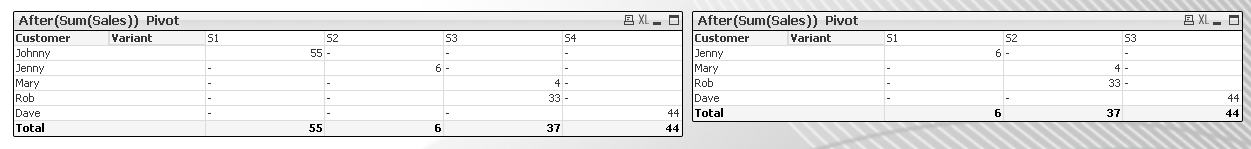6.First Function:

Syntax:  first( [ total ] expression [, offset [,n]] )

• Returns the value of expression evaluated with a pivot table’s dimension values as they appear in the first column of the current row segment in the pivot table. This function returns NULL in all chart types except pivot tables.
• If the table is one-dimensional or if the expression is preceded by the qualifier total, the current row segment is always equal to the entire row.

Sum(Sales):First(Sales):7.Last Function:

Syntax:  last( [ total ] expression [, offset [,n]] )

• Returns the value of expression evaluated with a pivot table’s dimension values as they appear in the last column of the current row segment in the pivot table. This function returns NULL in all chart types except pivot tables.
• If the table is one-dimensional or if the expression is preceded by the qualifier total, the current row segment is always equal to the entire row.

Sum(Sales):Last(Sales):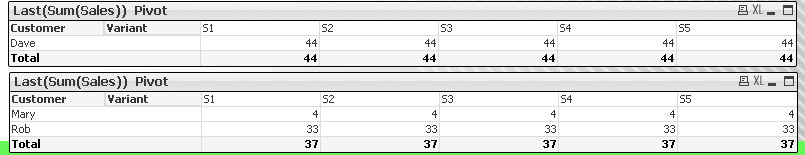8.Rowno Function:

Syntax:  RowNo( [ total ] )

• Returns the number of the current row within the current column segment in a table or, in the case of image charts, within the chart's straight table equivalent. The first row has number 1.
• If the chart is one-dimensional or if the qualifier total appears as argument, the current column segment is always equal to the entire column.

Rowno():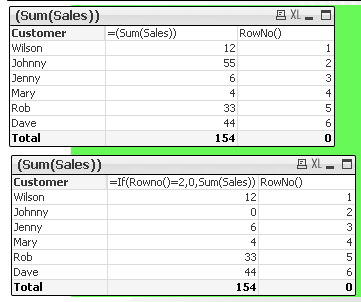9.No Of Rows Function:

Syntax:  NoOfRows( [ total ] )

• Returns the number of rows within the current column segment in a table or, in the case of image charts, within the chart's straight table equivalent.
• If the chart is one-dimensional or if the qualifier total appears as argument, the current column segment is always equal to the entire column.

NoOfRows():10.Column No Function:

Syntax: ColumnNo( [ total ] )

• Returns the number of the current row within the current row segment in a pivot table. The first column has number 1.
• If the table is one-dimensional or if the qualifier total appears as argument, the current row segment is always equal to the entire row.

Column No():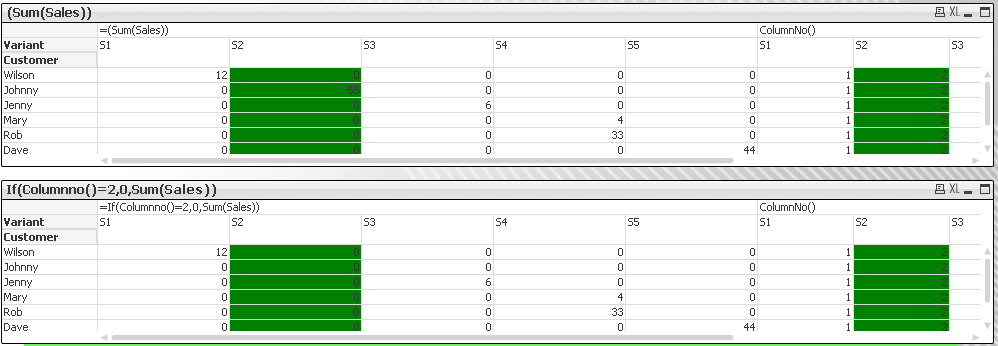11.No Of Columns Function:

Syntax: NoOfColumns( [ total ] )

•   Returns the number of columns in the current row segment of a pivot table.
•   If the table is one-dimensional or if the qualifier total appears as argument, the current row segment is always equal to the entire row.

No of Columns()12.Dimensionality() Function:

Syntax: dimensionality ( )

• Returns the number of dimension columns that have non-aggregation content, i.e. do not contain partial sums or collapse aggregates.A typical use is in attribute expressions, when you want to apply different cell formatting depending on aggregation level of data.
• This function is only available in charts. For all chart types except pivot tables it will return the number of dimensions in all rows except the total, which will be 0.

Example:

For a pivot table with three dimension columns to the left the following would be returned:

3 for all ordinary data cells

2 for 1st level partial sums and entries not expanded in the 3rd column

1st for 2nd level partial and entries only expanded in the 1st column.

0 for grand total cell.

Dimensionality():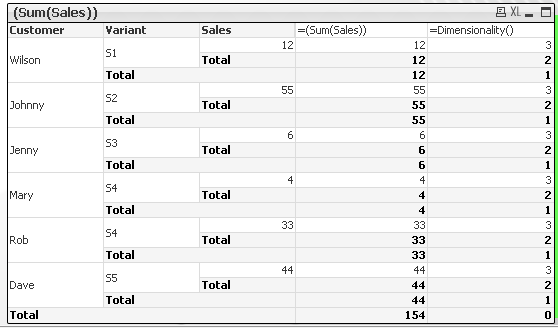Thus,These all above Functions will Come under Inter Record Functions Particularly at front end.

Please Find the Attached Complete(Inter-Record Functions.QVW) Application.

Hope this Helps in understanding these functions,

Thanks & Best Regards,

Hirish.V

Labels (8)

• ### Technical BriefCreator II

Very useful thank you!Master

Thank You Robert_Mika‌ .Partner

useful document..Thanks for sharingNot applicable

Very useful, thanks for sharing Harish.Partner

Harish thanks for sharing.Useful infoMaster II

thx a lot. nice work!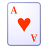Creator II

niceSpecialist II

HI hirishv7 :

Nice post, thanks for sharing

Joaquin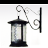Specialist

very useful ThankYou hirishv7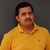Specialist III

Its very useful for beginners.

Version history
Last update:
‎2019-04-22 04:09 AM
Updated by:Contributors